#Interactive Real Analysis

Next | Previous | Glossary | Map

## 4.2. Convergence Tests

### Cauchy Condensation Test

Supposeis a decreasing sequence of positive terms. Then the series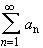converges if and only if the series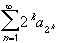converges.

This test is rather specialized, just as Abel's Convergence Test. The main purpose of the Cauchy Condensation test is to prove that the p-series converges if p > 1.

 Example 4.2.8:Use the Cauchy Condensation criteria to answer the following questions: In the sum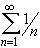, list the terms a4, ak, and a2k. Then show that this series (called the harmonic series) diverges. For which p does the series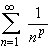converge or diverge ? (In addition to the p-Series test , recall the Geometric Series Test for this example)

Proof:

Assume thatconverges: We have

2k-1 a2k = a2k + a2k + a2k + ... + a2kbecause the sequence is decreasing. Hence, we have that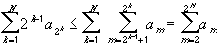Now the partial sums on the right are bounded, by assumption. Hence the partial sums on the left are also bounded. Since all terms are positive, the partial sums now form an increasing sequence that is bounded above, hence it must converge. Multiplying the left sequence by 2 will not change convergence, and hence the seriesconverges.

Assume thatconverges: We have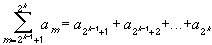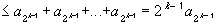Therefore, similar to above, we get: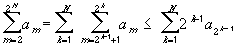But now the sequence of partial sums on the right is bounded, by assumption. Therefore, the left side forms an increasing sequence that is bounded above, and therefore must converge.Next | Previous | Glossary | Map